Open in App
Not now

# GroupBy and filter data in PySpark

• Last Updated : 19 Dec, 2021

In this article, we will Group and filter the data in PySpark using Python.

## Python3

 `# importing module ` `import` `pyspark ` ` `  `# importing sparksession from pyspark.sql module ` `from` `pyspark.sql ``import` `SparkSession ` ` `  `# creating sparksession and giving an app name ` `spark ``=` `SparkSession.builder.appName(``'sparkdf'``).getOrCreate() ` ` `  `# list  of student  data ` `data ``=` `[[``"1"``, ``"sravan"``, ``"IT"``, ``45000``], ` `        ``[``"2"``, ``"ojaswi"``, ``"CS"``, ``85000``], ` `        ``[``"3"``, ``"rohith"``, ``"CS"``, ``41000``], ` `        ``[``"4"``, ``"sridevi"``, ``"IT"``, ``56000``], ` `        ``[``"5"``, ``"bobby"``, ``"ECE"``, ``45000``], ` `        ``[``"6"``, ``"gayatri"``, ``"ECE"``, ``49000``], ` `        ``[``"7"``, ``"gnanesh"``, ``"CS"``, ``45000``], ` `        ``[``"8"``, ``"bhanu"``, ``"Mech"``, ``21000``] ` `        ``] ` ` `  `# specify column names ` `columns ``=` `[``'ID'``, ``'NAME'``, ``'DEPT'``, ``'FEE'``] ` ` `  `# creating a dataframe from the lists of data ` `dataframe ``=` `spark.createDataFrame(data, columns) ` ` `  `# display ` `dataframe.show() `

Output: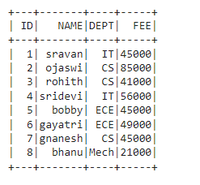In PySpark,  groupBy() is used to collect the identical data into groups on the PySpark DataFrame and perform aggregate functions on the grouped data. We have to use any one of the functions with groupby while using the method

Syntax: dataframe.groupBy(‘column_name_group’).aggregate_operation(‘column_name’)

Filter the data means removing some data based on the condition. In PySpark we can do filtering by using filter() and where() function

## Method 1: Using filter()

This is used to filter the dataframe based on the condition and returns the resultant dataframe

Syntax: filter(col(‘column_name’) condition )

filter with groupby():

dataframe.groupBy(‘column_name_group’).agg(aggregate_function(‘column_name’).alias(“new_column_name”)).filter(col(‘new_column_name’) condition )

where,

• dataframe is the input dataframe
• column_name_group is the column to be grouped
• column_name is the column that gets aggregated with aggregate operations
• aggregate_function is among the functions – sum(),min(),max() ,count(),avg()
• new_column_name is the column to be given from old column
• col is the function to specify the column on filter
• condition is to get the data from the dataframe using relational operators

## Python3

 `# importing module ` `import` `pyspark ` ` `  `# importing sparksession from pyspark.sql module ` `from` `pyspark.sql ``import` `SparkSession ` ` `  `#import col ` `from` `pyspark.sql.functions ``import` `col, ``sum` ` `  `# creating sparksession and giving an app name ` `spark ``=` `SparkSession.builder.appName(``'sparkdf'``).getOrCreate() ` ` `  `# list  of student  data ` `data ``=` `[[``"1"``, ``"sravan"``, ``"IT"``, ``45000``], ` `        ``[``"2"``, ``"ojaswi"``, ``"CS"``, ``85000``], ` `        ``[``"3"``, ``"rohith"``, ``"CS"``, ``41000``], ` `        ``[``"4"``, ``"sridevi"``, ``"IT"``, ``56000``], ` `        ``[``"5"``, ``"bobby"``, ``"ECE"``, ``45000``], ` `        ``[``"6"``, ``"gayatri"``, ``"ECE"``, ``49000``], ` `        ``[``"7"``, ``"gnanesh"``, ``"CS"``, ``45000``], ` `        ``[``"8"``, ``"bhanu"``, ``"Mech"``, ``21000``] ` `        ``] ` ` `  `# specify column names ` `columns ``=` `[``'ID'``, ``'NAME'``, ``'DEPT'``, ``'FEE'``] ` ` `  `# creating a dataframe from the lists of data ` `dataframe ``=` `spark.createDataFrame(data, columns) ` ` `  `# Groupby with DEPT  with sum() ` `# to get FEE greater than 56700 ` `dataframe.groupBy(``'DEPT'``).agg(``sum``( ` `  ``'FEE'``).alias(``"Total Fee"``)).``filter``( ` `  ``col(``'Total Fee'``) >``=` `56700``).show() `

Output: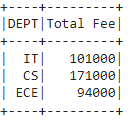## Python3

 `# importing module ` `import` `pyspark ` ` `  `# importing sparksession from pyspark.sql module ` `from` `pyspark.sql ``import` `SparkSession ` ` `  `#import col ` `from` `pyspark.sql.functions ``import` `col, ``sum` ` `  `# creating sparksession and giving an app name ` `spark ``=` `SparkSession.builder.appName(``'sparkdf'``).getOrCreate() ` ` `  `# list  of student  data ` `data ``=` `[[``"1"``, ``"sravan"``, ``"IT"``, ``45000``], ` `        ``[``"2"``, ``"ojaswi"``, ``"CS"``, ``85000``], ` `        ``[``"3"``, ``"rohith"``, ``"CS"``, ``41000``], ` `        ``[``"4"``, ``"sridevi"``, ``"IT"``, ``56000``], ` `        ``[``"5"``, ``"bobby"``, ``"ECE"``, ``45000``], ` `        ``[``"6"``, ``"gayatri"``, ``"ECE"``, ``49000``], ` `        ``[``"7"``, ``"gnanesh"``, ``"CS"``, ``45000``], ` `        ``[``"8"``, ``"bhanu"``, ``"Mech"``, ``21000``] ` `        ``] ` ` `  `# specify column names ` `columns ``=` `[``'ID'``, ``'NAME'``, ``'DEPT'``, ``'FEE'``] ` ` `  `# creating a dataframe from the lists of data ` `dataframe ``=` `spark.createDataFrame(data, columns) ` ` `  `# Groupby with DEPT  with sum() ` `# to get FEE greater than or equal to  ` `# 56700 and less than or equal to 100000 ` `dataframe.groupBy(``'DEPT'``).agg(``sum``( ` `  ``'FEE'``).alias(``"Total Fee"``)).``filter``( ` `    ``col(``'Total Fee'``) >``=` `56700``).``filter``( ` `  ``col(``'Total Fee'``) <``=` `100000``).show() `

Output: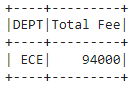## Method 2: Using where()

This is used to select  the dataframe based on the condition and returns the resultant dataframe

Syntax: where(col(‘column_name’) condition )

where with groupby():

dataframe.groupBy(‘column_name_group’).agg(aggregate_function(‘column_name’).alias(“new_column_name”)).where(col(‘new_column_name’) condition )

where,

• dataframe is the input dataframe
• column_name_group is the column to be grouped
• column_name is the column that gets aggregated with aggregate operations
• aggregate_function is among the functions – sum(),min(),max() ,count(),avg()
• new_column_name is the column to be given from old column
• col is the function to specify the column on where
• condition is to get the data from the dataframe using relational operators

## Python3

 `# importing module ` `import` `pyspark ` ` `  `# importing sparksession from pyspark.sql module ` `from` `pyspark.sql ``import` `SparkSession ` ` `  `#import col ` `from` `pyspark.sql.functions ``import` `col, ``sum` ` `  `# creating sparksession and giving an app name ` `spark ``=` `SparkSession.builder.appName(``'sparkdf'``).getOrCreate() ` ` `  `# list  of student  data ` `data ``=` `[[``"1"``, ``"sravan"``, ``"IT"``, ``45000``], ` `        ``[``"2"``, ``"ojaswi"``, ``"CS"``, ``85000``], ` `        ``[``"3"``, ``"rohith"``, ``"CS"``, ``41000``], ` `        ``[``"4"``, ``"sridevi"``, ``"IT"``, ``56000``], ` `        ``[``"5"``, ``"bobby"``, ``"ECE"``, ``45000``], ` `        ``[``"6"``, ``"gayatri"``, ``"ECE"``, ``49000``], ` `        ``[``"7"``, ``"gnanesh"``, ``"CS"``, ``45000``], ` `        ``[``"8"``, ``"bhanu"``, ``"Mech"``, ``21000``] ` `        ``] ` ` `  `# specify column names ` `columns ``=` `[``'ID'``, ``'NAME'``, ``'DEPT'``, ``'FEE'``] ` ` `  `# creating a dataframe from the lists of data ` `dataframe ``=` `spark.createDataFrame(data, columns) ` ` `  ` `  `# Groupby with DEPT  with sum() to get ` `# FEE greater than or equal to  56700 ` `dataframe.groupBy(``'DEPT'``).agg(``sum``( ` `  ``'FEE'``).alias(``"Total Fee"``)).where( ` `  ``col(``'Total Fee'``) >``=` `56700``).show() `

Output:## Python3

 `# importing module ` `import` `pyspark ` ` `  `# importing sparksession from pyspark.sql module ` `from` `pyspark.sql ``import` `SparkSession ` ` `  `#import col ` `from` `pyspark.sql.functions ``import` `col, ``sum` ` `  `# creating sparksession and giving an app name ` `spark ``=` `SparkSession.builder.appName(``'sparkdf'``).getOrCreate() ` ` `  `# list  of student  data ` `data ``=` `[[``"1"``, ``"sravan"``, ``"IT"``, ``45000``], ` `        ``[``"2"``, ``"ojaswi"``, ``"CS"``, ``85000``], ` `        ``[``"3"``, ``"rohith"``, ``"CS"``, ``41000``], ` `        ``[``"4"``, ``"sridevi"``, ``"IT"``, ``56000``], ` `        ``[``"5"``, ``"bobby"``, ``"ECE"``, ``45000``], ` `        ``[``"6"``, ``"gayatri"``, ``"ECE"``, ``49000``], ` `        ``[``"7"``, ``"gnanesh"``, ``"CS"``, ``45000``], ` `        ``[``"8"``, ``"bhanu"``, ``"Mech"``, ``21000``] ` `        ``] ` ` `  `# specify column names ` `columns ``=` `[``'ID'``, ``'NAME'``, ``'DEPT'``, ``'FEE'``] ` ` `  `# creating a dataframe from the lists of data ` `dataframe ``=` `spark.createDataFrame(data, columns) ` ` `  ` `  `# Groupby with DEPT  with sum() to get ` `# FEE greater than or equal to  56700 ` `# and less than or equal to 100000 ` `dataframe.groupBy(``'DEPT'``).agg(``sum``( ` `  ``'FEE'``).alias(``"Total Fee"``)).where( ` `    ``col(``'Total Fee'``) >``=` `56700``).where( ` `  ``col(``'Total Fee'``) <``=` `100000``).show() `

Output:## Method 3: Using Window Function

The window function is used for partitioning the columns in the dataframe

Syntax: Window.partitionBy(‘column_name_group’)

where, column_name_group is the column that contains multiple values for partition

We can partition the data column that contains group values and then use the aggregate functions like min(), max, etc to get the data. In this way, we are going to filter the data from the PySpark DataFrame with where clause.

Syntax: dataframe.withColumn(‘new column’, functions.max(‘column_name’).over(Window.partitionBy(‘column_name_group’))).where(functions.col(‘column_name’) == functions.col(‘new_column_name’))

where,

• dataframe is the input dataframe
• column_name_group is the column to be partitioned
• column_name is to get the values with grouped column
• new_column_name is the new filtered column

## Python3

 `# importing module ` `import` `pyspark ` ` `  `# importing sparksession from pyspark.sql module ` `from` `pyspark.sql ``import` `SparkSession ` ` `  `#import functions ` `from` `pyspark.sql ``import` `functions as f ` ` `  `# import window module ` `from` `pyspark.sql ``import` `Window ` ` `  `# creating sparksession and giving an app name ` `spark ``=` `SparkSession.builder.appName(``'sparkdf'``).getOrCreate() ` ` `  `# list  of student  data ` `data ``=` `[[``"1"``, ``"sravan"``, ``"IT"``, ``45000``], ` `        ``[``"2"``, ``"ojaswi"``, ``"CS"``, ``85000``], ` `        ``[``"3"``, ``"rohith"``, ``"CS"``, ``41000``], ` `        ``[``"4"``, ``"sridevi"``, ``"IT"``, ``56000``], ` `        ``[``"5"``, ``"bobby"``, ``"ECE"``, ``45000``], ` `        ``[``"6"``, ``"gayatri"``, ``"ECE"``, ``49000``], ` `        ``[``"7"``, ``"gnanesh"``, ``"CS"``, ``45000``], ` `        ``[``"8"``, ``"bhanu"``, ``"Mech"``, ``21000``] ` `        ``] ` ` `  `# specify column names ` `columns ``=` `[``'ID'``, ``'NAME'``, ``'DEPT'``, ``'FEE'``] ` ` `  `# creating a dataframe from the lists of data ` `dataframe ``=` `spark.createDataFrame(data, columns) ` ` `  ` `  `# display ` `dataframe.withColumn(``'FEE max'``, f.``max``(``'FEE'``).over( ` `    ``Window.partitionBy(``'DEPT'``))).where( ` `  ``f.col(``'FEE'``) ``=``=` `f.col(``'FEE max'``)).show() `

Output: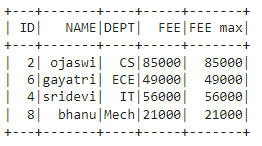## Method 4: Using join

We can filter the data with aggregate operations using leftsemi join, This join will return the left matching data from dataframe1 with the aggregate operation

Syntax: dataframe.join(dataframe.groupBy(‘column_name_group’).agg(f.max(‘column_name’).alias(‘new_column_name’)),on=’FEE’,how=’leftsemi’)

## Python3

 `# importing module ` `import` `pyspark ` ` `  `# importing sparksession from pyspark.sql module ` `from` `pyspark.sql ``import` `SparkSession ` ` `  `#import functions ` `from` `pyspark.sql ``import` `functions as f ` ` `  `# import window module ` `from` `pyspark.sql ``import` `Window ` ` `  `# creating sparksession and giving an app name ` `spark ``=` `SparkSession.builder.appName(``'sparkdf'``).getOrCreate() ` ` `  `# list  of student  data ` `data ``=` `[[``"1"``, ``"sravan"``, ``"IT"``, ``45000``], ` `        ``[``"2"``, ``"ojaswi"``, ``"CS"``, ``85000``], ` `        ``[``"3"``, ``"rohith"``, ``"CS"``, ``41000``], ` `        ``[``"4"``, ``"sridevi"``, ``"IT"``, ``56000``], ` `        ``[``"5"``, ``"bobby"``, ``"ECE"``, ``45000``], ` `        ``[``"6"``, ``"gayatri"``, ``"ECE"``, ``49000``], ` `        ``[``"7"``, ``"gnanesh"``, ``"CS"``, ``45000``], ` `        ``[``"8"``, ``"bhanu"``, ``"Mech"``, ``21000``] ` `        ``] ` ` `  `# specify column names ` `columns ``=` `[``'ID'``, ``'NAME'``, ``'DEPT'``, ``'FEE'``] ` ` `  `# creating a dataframe from the lists of data ` `dataframe ``=` `spark.createDataFrame(data, columns) ` ` `  ` `  `# display ` `dataframe.join(dataframe.groupBy(``'DEPT'``).agg( ` `    ``f.``max``(``'FEE'``).alias(``'FEE'``)), on``=``'FEE'``,  ` `               ``how``=``'leftsemi'``).show() `

Output: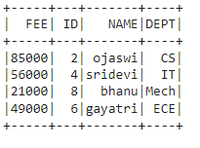My Personal Notes arrow_drop_up
Related Articles